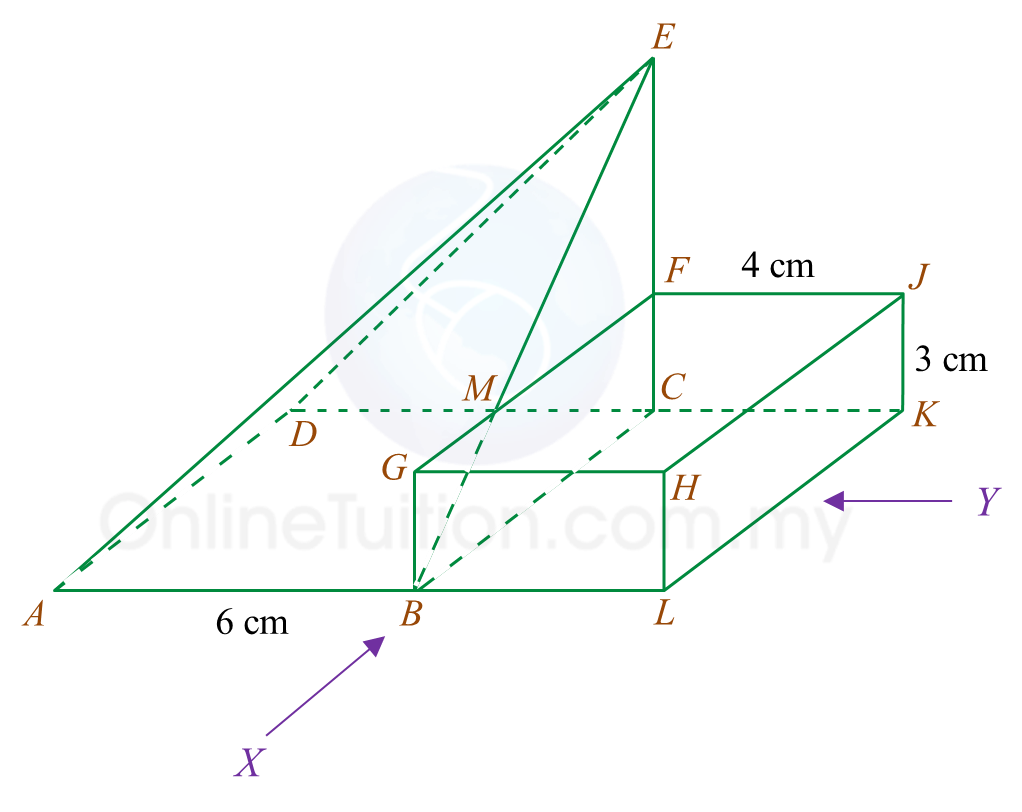# 7.3.4 Plans and Elevations, PT3 Focus Practice

Question 5:
You are not allowed to use graph paper to answer this question.

(a)
Diagram 15.1 shows a pyramid with rectangular base ABCD on a horizontal plane. Vertex E is vertically above C. Triangles BCE and DCE are vertical planes. Triangles ABE and ADE are inclined planes.Diagram 5.1

Draw to full scale, the plan of the solid.

(b)
Another solid cuboid with rectangle base BLKC is joined to the pyramid in Diagram 5.1 at the vertical plane BCFM. The composite solid is as shown in Diagram 5.2. The base ABLKCD lies on a horizontal planeDiagram 5.2

Draw to full scale,
(i) the elevation of the composite solid on a vertical plane parallel to ABL as viewed from X.

(ii)
the elevation of the composite solid on a vertical plane parallel to LK as viewed from Y.

Solution:

(a)(b)(i)(b)(ii)